# Distance Measure Types in Machine Learning

## Distance Measure Types in Machine Learning

In this article, I am going to discuss Distance Measure Types in Machine Learning with Examples. Please read our previous article where we discussed Similarity Metrics in Machine Learning with Examples.

##### Distance Measure Types in Machine Learning

Several machine learning techniques include distance measures as a significant component. These distance metrics are used to calculate the similarity between data points in both supervised and unsupervised learning.

Whether it’s for classification or clustering, and efficient distance measure improves the performance of our machine learning model. In machine learning, there are four different types of distance metrics.

1. Euclidean Distance
2. Manhattan Distance
3. Minkowski Distance
4. Hamming Distance
##### Euclidean Distance in Machine Learning

The smallest distance between two points is known as the Euclidean Distance. This distance metric is used by most machine learning algorithms, including K-Means, to measure the similarity of observations. Assume we have the following two points: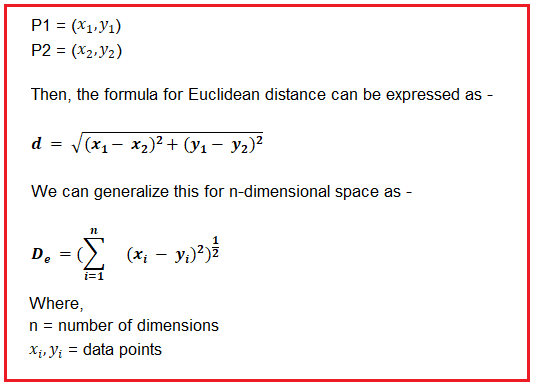Euclidean distance can be visualized graphically as –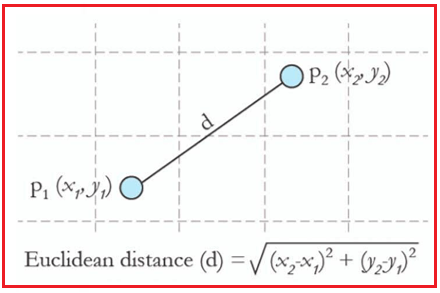##### Manhattan Distancein Machine Learning

The Manhattan Distance is the total difference between two places in all dimensions. The term “Manhattan Distance” is frequently used to refer to the distance between two city blocks. Let’s consider two points – A = () and B = (). Manhattan Distance can be expressed graphically as follows: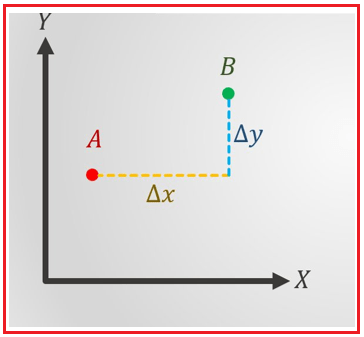Because the following illustration is two-dimensional, we’ll use the total of absolute distances in both the x and y axes to calculate Manhattan Distance.

In a two-dimensional space, the Manhattan distance is given as: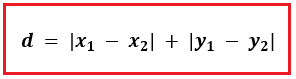For an n-dimensional space, we can generalize the same as: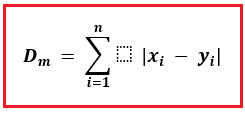Where,

n = number of dimensions

= data points

##### Minkowski Distance in Machine Learning

The generalized form of the Euclidean and Manhattan Distances is the Minkowski Distance. You can express the Minkowski distance as –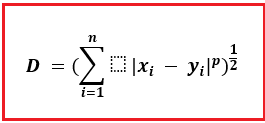The order of the norm is represented by p.

When an order(p) is 1, Manhattan Distance is represented, and when order(p) is 2 in the above formula, Euclidean Distance is represented.

##### Hamming Distance in Machine Learning

The Hamming Distance is a measurement of how similar two strings of the same length are. The Hamming Distance is the number of spots where the corresponding characters differ between two strings of the same length.

Let’s look at an example to better comprehend the notion. Let’s pretend we’ve got two strings:

“Codenet” and “Dotnets”

We can determine the Hamming Distance because the lengths of these strings are equivalent. We’ll match the strings one by one, character by character. If we look closely – three characters are distinct, while four characters are similar.

As a result, the Hamming Distance will be 3. The greater the Hamming Distance between two strings, the more different those strings will be (and vice versa). Only when we have strings or arrays of the same length does Hamming distance operate.

In the next article, I am going to discuss Creating Predictive Models in Machine Learning with Examples. Here, in this article, I try to explain Distance Measure Types in Machine Learning with Examples. I hope you enjoy this Distance Measure Types in Machine Learning with Examples article.